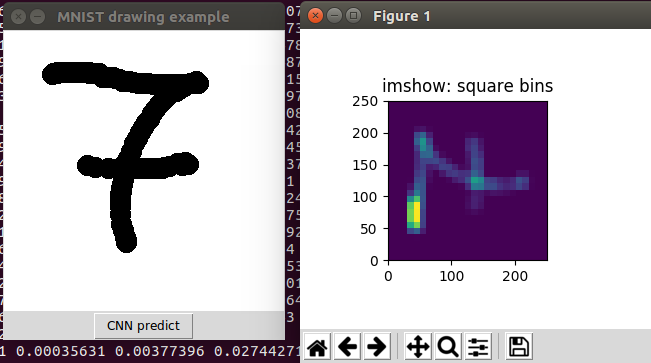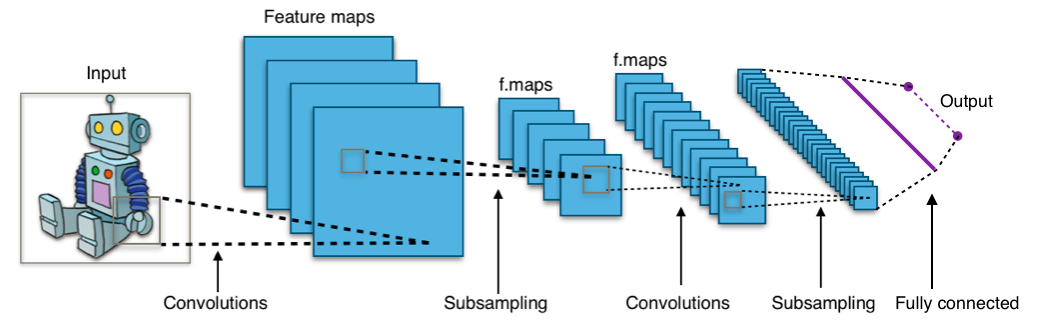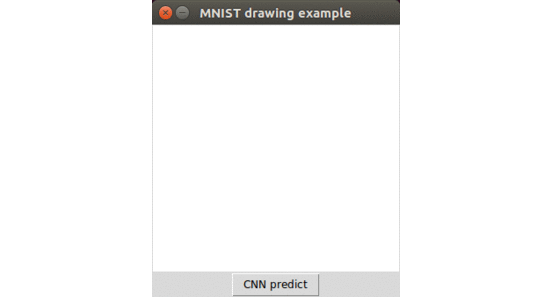# MNIST with a CNN in TensorFlow

by Daniel Pollithy — on  ,  ,  ,

## Quickstart to TensorFlow

I decided to build the classic MNIST hello world digit recognition with a convolutional neural network in tensorflow. And then I wrote a small Tkinter user interface to draw numbers, sample them down and feed them into the estimator.### Installation

The installation is really straight forward if you have a virtualenv setup: `pip install tensorflow`

You are also going to need `numpy` and `matplotlib`.

### Test the installation

You can run this little script to check whether tensorflow was installed completely.

``````import tensorflow as tf
hello = tf.constant("Let's go, TensorFlow!")
sess = tf.Session()
print(sess.run(hello))
``````

### Detecting digits

The introduction to tensorflow uses softmax regression which is a good starter for non-binary classification but I also found a good tutorial on how to use a convolutional neural network for this exercise.CC BY-SA 4.0 (user Aphex34 on wikipedia)

Every image in MNIST has 28x28 grayscale pixels (from 0.0 to 1.0) where 1.0 means black.

The setup of the CNN is:

• Convolution layer 1: from 28x28x1 to 28x28x32 because we apply 32 filter
• Pooling layer 1: from 28x28x32 to 14x14x32 with a pool size of 2x2
• Convolution layer 2: from 14x14x32 to 14x14x64
• Pooling layer 2: from 14x14x64 to 7x7x64 with pool size 2x2
• Dense layer: from 7x7x64 aligned to vectors to 1024x1
• Logits layer: 1024x1 to 10x1

Copy and paste the example code (Code tensorflow) into a file called cnn_mnist.py and run the python script.

The training took about one hour on Intel® Core™ i5-3320M CPU @ 2.60GHz × 4 (no gpu involved).

And the evaluation resulted in `{'loss': 0.10442939, 'global_step': 20000, 'accuracy': 0.9688}`.

## Drawing my own numbers

I patched the following user interface together:

``````import numpy as np
import tensorflow as tf
from cnn_mnist import cnn_model_fn
import matplotlib.pyplot as plt
import Tkinter as tk
import tkMessageBox

class Gui(object):
def __init__(self):
self.root = tk.Tk()
self.root.title('MNIST drawing example')
self.root.resizable(0,0)

self.c = tk.Canvas(self.root, bg="white", width=280, height=280)

self.c.configure(cursor="crosshair")
self.c.pack()

self.pen_width = 20
self.c.bind("<B1-Motion>", self.paint )

self.button_predict = tk.Button(self.root, text="CNN predict", command=self.predict_callback)
self.button_predict.pack()

self.points = set()

self.mnist_classifier = tf.estimator.Estimator(model_fn=cnn_model_fn, model_dir="./mnist_convnet_model")

def paint(self, event ):
x1, y1 = ( event.x - self.pen_width ), ( event.y - self.pen_width )
x2, y2 = ( event.x + self.pen_width ), ( event.y + self.pen_width )
self.c.create_oval( x1, y1, x2, y2, fill = "black" )

for x_ in range(x1,x2):
for y_ in range(y1,y2):

def get_histogram_data(self, show=True):

xedges = list(range(0, 290, 10))
yedges = list(range(0, 290, 10))

points = [np.array([x,y], dtype=np.float32) for x,y in self.points]
points = np.asarray(points)
points = points.reshape(-1, 2)
x = points[:,0]
y = points[:,1]
H, xedges, yedges = np.histogram2d(x, y, bins=(xedges, yedges))
H /= 100.0

if show:
fig = plt.figure(figsize=(7, 3))
ax = fig.add_subplot(131, title='imshow: square bins')
plt.imshow(H, interpolation='nearest', origin='low',
extent=[xedges, xedges[-1], yedges, yedges[-1]])
plt.show()

H = H.astype(np.float32)

return H

def predict_callback(self):
data = self.get_histogram_data(show=True)

eval_input_fn = tf.estimator.inputs.numpy_input_fn(
x={"x": data },
num_epochs=1,
shuffle=False)

eval_results = self.mnist_classifier.predict(input_fn=eval_input_fn)

result = list(eval_results)['classes']

tkMessageBox.showinfo("Mnist CNN reports", "Your drawing is a {}".format(result))

if __name__ == '__main__':
g = Gui()
g.root.mainloop()
``````

Where the few interesting lines are:

`self.mnist_classifier = tf.estimator.Estimator(model_fn=cnn_model_fn, model_dir="./mnist_convnet_model")` This loades the trained model from the directory “mnist_convnet_model” (it might be in “/temp/mnist_convnet_model” on your machine)

`def get_histogram_data(self, show=True):` samples the 280x280 pixels on the canvas down to 28x28 buckets containing values from 0.0 to 1.0.

``````    eval_input_fn = tf.estimator.inputs.numpy_input_fn(
x={"x": data },
num_epochs=1,
shuffle=False)

eval_results = self.mnist_classifier.predict(input_fn=eval_input_fn)
``````

And this part wraps the 1d-numpy array called `data` into a function that is callable to deliver the format for the `.predict(...)` method of the estimator.

## Final resultThis was fun. Although I have to say that it really doesn’t work perfectly. The digits are easy mistaken and I have the feeling that my data is far too clean. I guess I would have to downsample it properly to improve the results.